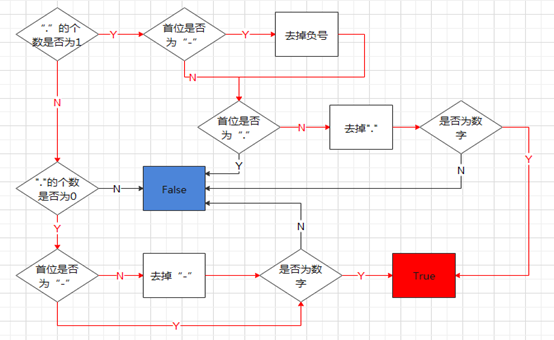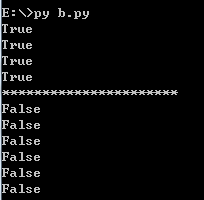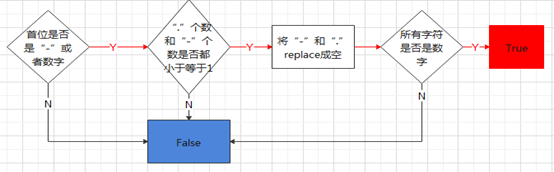• python判断字符串是数字
千次阅读
2021-03-18 08:17:52

如何使用python判断字符串是纯数字

发布时间：2020-11-09 11:28:39

来源：亿速云

阅读：109

作者：小新

小编给大家分享一下如何使用python判断字符串是纯数字，希望大家阅读完这篇文章后大所收获，下面让我们一起去探讨吧！

Isdigit()方法

检测字符串是否只由数字组成

语法：1 str.isdigit()

参数：

返回值：

如果字符串只包含数字，则返回True，否则返回False。

实例：

以下实例展示了isdigit()方法的实例：str = '123456'

print(str.isdigit()) #True

str = "耿雨飞"

print(str.isdigit()) #False

Isnumeric()方法

检测字符串是否只由数字组成,这种方法只是针对Unicode对象。

注：定义一个字符串为Unicode，只需要在字符串前添加'u'前缀即可。

语法：1 str.isnumeric()

参数：

返回值：

如果字符串只包含数字，则返回True，否则返回False。str = "runoob2016"

print (str.isnumeric()) #False

str = "23443434"

print (str.isnumeric()) #True

看完了这篇文章，相信你对如何使用python判断字符串是纯数字有了一定的了解，想了解更多相关知识，欢迎关注亿速云行业资讯频道，感谢各位的阅读！

更多相关内容
• str.isdigit() # 判断字符串是否为纯数字，有任何的字母、标点符号（包括空格）都会返回False ———————————————————————————————————————————— str.isalpha() # 判断...

使用python字符串自带的函数即可实现：
注意：str.isdigit()， 带负号的数字也会返回False

str.isdigit() # 判断字符串是否为纯数字，有任何的字母、标点符号（包括空格、负号）都会返回False
————————————————————————————————————————————
str.isalpha() # 判断字符串是否为纯字母（不区分大小写），有任何的数字、标点符号都会返回False
————————————————————————————————————————————
str.isalnum（） # 判断字符串是否为数字和字母的的混合，有任何的标点符号都会返回False

str_1 = "123"
str_2 = "Abc"
str_3 = "123Abc"
str_4 = "-123"

#用isdigit函数判断是否数字
print(str_1.isdigit())
Ture
print(str_2.isdigit())
False
print(str_3.isdigit())
False
print(str_4.isdigit())
False

#用isalpha判断是否字母
print(str_1.isalpha())
False
print(str_2.isalpha())
Ture
print(str_3.isalpha())
False

#isalnum判断是否数字和字母的组合
print(str_1.isalnum())
Ture
print(str_2.isalnum())
Ture
print(str_1.isalnum())
Ture

注意：如果字符串中含有除了字母或者数字之外的字符，比如空格，也会返回False

展开全文python 字符串
• ## Python判断字符串是数字还是字母

万次阅读 多人点赞 2018-12-03 17:07:13
严格解析：有除了数字或者字母外的符号（空格，分号,etc.）都会False isalnum()必须是数字和字母的混合 ...#用isdigit函数判断是否数字 print(str_1.isdigit()) Ture print(str_2.isdigit()) False ...
严格解析：有除了数字或者字母外的符号（空格，分号,etc.）都会False
isalnum()必须是数字和字母的混合
isalpha()不区分大小写

str_1 = "123"
str_2 = "Abc"
str_3 = "123Abc"

#用isdigit函数判断是否数字
print(str_1.isdigit())
Ture
print(str_2.isdigit())
False
print(str_3.isdigit())
False

#用isalpha判断是否字母
print(str_1.isalpha())
False
print(str_2.isalpha())
Ture
print(str_3.isalpha())
False

#isalnum判断是否数字和字母的组合
print(str_1.isalnum())
Ture
print(str_2.isalnum())
Ture
print(str_1.isalnum())
Ture
注意：如果字符串中含有除了字母或者数字之外的字符，比如空格，也会返回False

str为字符串s为字符串

str.isalnum() 所有字符都是数字或者字母

str.isalpha() 所有字符都是字母

str.isdigit() 所有字符都是数字

str.isspace() 所有字符都是空白字符、t、n、r

检查字符串是数字/浮点数方法

#float部分
>> float('Nan')
nan
>> float('Nan')
nan
>> float('nan')
nan
>> float('INF')
inf
>> float('inf')
inf
>> float('-INF')
inf
>> float('-inf')
inf

#第一种：最简单
def is_number(str):
try:
# 因为使用float有一个例外是'NaN'
if str=='NaN':
return False
float(str)
return True
except ValueError:
return False
float例外示例
>>> float('NaN')
nan

#使用complex()
def is_number(s):
try:
complex(s) # for int, long, float and complex
except ValueError:
return False
return True

#综合1
def is_number(s):
try:
float(s) # for int, long and float
except ValueError:
try:
complex(s) # for complex
except ValueError:
return False
return True

#综合2-还是无法完全识别
def is_number(n):
is_number = True
try:
num = float(n)
# 检查 "nan"
is_number = num == num  # 或者使用 math.isnan(num)
except ValueError:
is_number = False
return is_number
>>> is_number('Nan')
False
>>> is_number('nan')
False
>>> is_number('123')
True
>>> is_number('-123')
True
>>> is_number('-1.12')
True
>>> is_number('abc')
False
>>> is_number('inf')
True

#第二种：只能判断是整数

#使用isnumeric()
# str必须是uniconde模式
>>> str = u"345"
>>> str.isnumeric()True
#http://www.tutorialspoint.com/python/string_isnumeric.htm
#http://docs.python.org/2/howt...

#使用isdigit()
#https://docs.python.org/2/lib...
>>> str = "11"
>>> print str.isdigit()
True
>>> str = "3.14"
>>> print str.isdigit()
False
>>> str = "aaa"
>>> print str.isdigit()
False

#使用int()
def is_int(str):
try:
int(str)
return True
except ValueError:
return False

#第三种：使用正则(最安全方法)
import re
def is_number(num):
pattern = re.compile(r'^[-+]?[-0-9]\d*\.\d*|[-+]?\.?[0-9]\d*$') result = pattern.match(num) if result: return True else: return False >>>: is_number('1') True >>>: is_number('111') True >>>: is_number('11.1') True >>>: is_number('-11.1') True >>>: is_number('inf') False >>>: is_number('-inf') False https://www.cnblogs.com/wangboqi/p/7455240.html 展开全文python • 判断字符串是否是数字（包含负数和小数） 算法1： 1、分小数和整数：如果小数点个数为1，则可能是小数。如小数点个数为0，则可能是整数。小数点个数非0也非1，那么就不是数字。 2、如果是小数，再分正数和负数的情况... #### 判断字符串是否是数字（包含负数和小数） 算法1： 1、分小数和整数：如果小数点个数为1，则可能是小数。如小数点个数为0，则可能是整数。小数点个数非0也非1，那么就不是数字。 2、如果是小数，再分正数和负数的情况：如果首位为负号，则可能是负小数。如果首位不为负号，则可能是正小数。 1）如果首位为负号，则去掉负号。 2）如果首位不为负号；或者是首位为负号，去掉首位负号之后。则判断是否为是否为“.”，如果是，那么就不是数字，如果不是，就去掉"." 3）再判断剩下字符是否都在"0123456789"内，如果不都在则不是数字，否则就是数字 3、如果是整数，也同样分正数和负数的情况。如果首位为负号，则可能是负整数，如果首位不为负号，则可能是正整数。 1）如果首位诶负号，则去掉负号。 2）如果首位不为负号；或者是首位为负号，去掉首位负号之后。判断剩下字符是否都在"0123456789"内，如果不都在则不是数字，否则就是数字def is_number(s): if s.count(".")==1: #小数的判断 if s=="-": s=s[1:] if s==".": return False s=s.replace(".","") for i in s: if i not in "0123456789": return False else: #这个else与for对应的 return True elif s.count(".")==0: #整数的判断 if s=="-": s=s[1:] for i in s: if i not in "0123456789": return False else: return True else: return False print(is_number("1334345345")) print(is_number("1.12344565")) print(is_number("-14344343")) print(is_number("-1.123456")) print("**********************") print(is_number("abc")) print(is_number("1.32.2")) print(is_number(".2344")) print(is_number("1234a")) print(is_number("--1.1")) print(is_number("--1abc"))算法2： 1、判断首位是否是负号或者数字，如果不是，则不是数字。如果是，则可能是数字。 2、判断".“和”-"的个数是否都小于等于1，如果不是，则不是数字，如果是，则可能是数字。 3、去掉".“和”-" 。 4、判断剩下的字符是否都在"0123456789"内，如果不都在则不是数字，否则就是数字''' 遇到问题没人解答？小编创建了一个Python学习交流QQ群：778463939 寻找有志同道合的小伙伴，互帮互助,群里还有不错的视频学习教程和PDF电子书！ ''' def is_number(s): if s=="-" or s in "0123456789": if s.count(".")<=1 and s.count("-")<=1: s=s.replace("-","").replace(".","") #print (s) for i in s: if i not in "0123456789": return False else: #这个else与for对应 return True else: return False else: return False print(is_number("1334345345")) print(is_number("1.12344565")) print(is_number("-14344343")) print(is_number("-1.123456")) print("**********************") print(is_number("abc")) print(is_number("1.32.2")) print(is_number(".2344")) print(is_number("1234a")) print(is_number("--1.1")) print(is_number("--1abc"))展开全文编程语言 python • str = raw_input("please input the number:") ...str为字符串 str.isalnum() 所有字符都是数字或者字母 str.isalpha() 所有字符都是字母 str.isdigit() 所有字符都是数字 str.islower() 所有字符都是小写...字符串 正则表达式 python • 本文给大家带来三种方法基于Python判断字符串是否为字母或者数字(浮点数),非常不错，具有一定的参考借鉴价值，需要的朋友可以参考下 • 这学期在学习编译原理，最近的上机作业就是做一个简单的词法分析器，在做的过程中，突然有个需求就是判断一个字符串是否为合法的标示符，因为我是用python语言做的，做的是Python的词法分析器，于是下面分享以下怎样... • 复制代码 代码如下:# -*- coding: cp936 -*-import re s1 = ‘adkkdk’s2 = ‘abc123efg’ an = re.search(‘^[a-z]+$’, s1)if an: print ‘s1:’, an.group(), ...1. 正则表达式不是python的一部分，利用时需要引用
•python
• isdigit函数判断是否数字 print(str_1.isdigit()) Ture print(str_2.isdigit()) False print(str_3.isdigit()) False isalpha判断是否字母 print(str_1.isalpha()) False print(str_2.isalpha()) Ture print(str_...python
• 判断字符串的数据类型 a='123' print(a.isdigit()) # 是否是数值 b='abc' print(b.isalpha()) # 是否是字母 c=' ' print(c.isspace()) # 是否是空格 函数 说明 isdigit 数字 isalpha 字母 isspace ...python
• #用isdigit函数判断是否数字 print(str_1.isdigit()) Ture print(str_2.isdigit()) False print(str_3.isdigit()) False #用isalpha判断是否字母 print(str_1.isalpha()) False print(str_2.isalpha()) Ture ...python
• 前言 ...判断字符串是否为数字（整形） 这个直接调用python的库就可以了哈，假设str为字符串 str.isdigit() 判断所有字符都是数字（整形） 返回值 True：该字符串都为数字；False：该字符串不...Python 判断字符串 浮点数
• 如下所示： x = 3 print(x+"nihao") ... 您可能感兴趣的文章:解决Python中字符串和数字拼接报错的方法Python实现字符串格式化输出的方法详解python 字符串和整数的转换方法python判断字符串是否纯数字的方法
• 判断字符串 s.isalnum() #所有字符都是数字或者字母 s.isalpha() #所有字符都是字母 s.isdigit() #所有字符都是数字 s.islower() #所有字符都是小写 s.isupper() #所有字符都是大写 s.istitle() #所有单词都是首...
• 1.用def定义四个函数，分别是用来判断汉字，数字，英语或其他（如下列代码所示） 2.最前面添加一个输入语句。最后面添加一个选择和输出同时的语句即可 def is_chinese(uchar): """判断一个unicode是否是汉字""" if...python
• 本文实例讲述了python判断字符串是否纯数字的方法。分享给大家供大家参考。具体如下： 判断的代码如下,通过异常判断不能区分前面带正负号的区别,正则表达式可以根据自己需要比较灵活的写,通过isdigit方法用来判断...
• Python3 基础语法-用python判断字符串是否全是数字python
• 利用Python自带的numerric方法轻松帮你判断字符串是否含有数字，涵盖范围广，谁用谁知道大数据 python
• Python的string库有isdigit()方法： import string string = "a1sjdih" if string.isdigit(): print(string) else: print("ERROR")python
• 使用正则表达式 很简单具体如下 str_content = "hello886" str_pattern = re.compile(r".*[0-9]\$... print("是以数字结尾,内容为:%s" % str_content) else: print("不是以数字结尾,内容为:%s" % str_content) ...
• 因工作原因，很久没有学习python知识了，感觉都快忘记了，前天看到一个练习题，如何将字符串中的数字提取出来，然后求和呢？下面我来解释一下如何通过python代码来实现。 题目：字符串43…3y2.f67se2.666. 将其中的...
• Python 数字类型与字符串作者：无名先生1.数字类型简介Python Number 数据类型用于存储数值。数据类型是不允许改变的,这就意味着如果改变 Number 数据类型的值，将重新分配内存空间。将数字赋予变量：X = 1Y = 10...
• Python 判断字符串是否为数字以下实例通过创建自定义函数 is_number() 方法来判断字符串是否为数字：#-*-coding:UTF-8-*-#Filename:test.pydefis_number(s):try:float(s)returnTrueexceptValueError:passtry:...
• 主要介绍了python通过自定义isnumber函数判断字符串是否为数字的方法,涉及Python操作字符串判断的相关技巧,需要的朋友可以参考下
• 一、判断数字 str.isnumeric() ReturnTrueif all characters in the string are numeric characters, and there is at least one character,Falseotherwise. Numeric characters include digit characters, and ...机器学习 python...